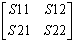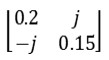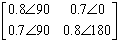# Basic network theory

To be able to grasp the concepts on many of our pages, there are a bunch of basic terms used almost universally throughout the Microwaves101 website that  need to clearly defined and understood. The performance and operation of most microwave objects are commonly described using network parameters determined by measurements or theory or both. Here is a clickable index that provides you with detailed information on how network parameters are defined and the properties associated with microwave networks.

Click here to go to our main page on the fundamental sets of network parameters including the definitions and interrelations between
S-parameters, Z-parameters, Y-parameters, ABCD parameters, and T-parameters.

Passive versus active devices

Unilateral versus non-unilateral devices

Reciprocal versus non-reciprocal devices

Isotropy (separate page)

Lossless networks

Matched networks

Special properties of three-port networks

Dispersion (separate page)

### Passive devices versus active devices

passive device contains no source that could add energy to your signal, so the power output at the ports is lesser than or equal to the power fed into the ports. The first law thermodynamics, conservation of energy, implies that a passive device can't oscillate. An active device is one in which an external energy source is somehow contributing to the magnitude of one or more responses.

The important properties of a passive network are:

• whether it is reciprocal or non-reciprocal
• whether it is lossy or lossless

### Unilateral versus non-unilateral devices

When we are discussing unilateral devices, we usually mean ideal amplifiers or other active devices. Two-port S-parameters almost always use the convention that the S21 power transfer is in the forward direction (from input to output, the direction of gain) and S12 is the reverse-isolation direction. A unilateral device has the property that S12=0. In practice, this is impossible to reach exactly, but it can be used as an approximation in some cases and sure makes network analysis easier.

### Properties of reciprocal and non-reciprocal networks

A reciprocal network is one in which the transmission of a signal between any two ports does not depend on the direction of propagation- input and output ports are interchangeable (scattering parameter S21=S12, S13=S31, etc.) A network is known to be reciprocal if it is passive and contains only isotropic materials. Examples of reciprocal networks include cables and standard transmission lines, attenuators, and the common passive power splitters and couplers. Two classic examples of non-reciprocal networks are RF amplifiers and isolators. In both cases, scattering parameter S21 is much different from S12. In fact, almost all active circuits are non-reciprocal since transistors are inherently non-reciprocal devices.

Anisotropic materials have different electrical properties  depending on the direction a signal propagates through them. One example of an anisotropic material is the class of materials known as ferrites, from which circulators and isolators are made. Two classic examples of non-reciprocal networks are RF amplifiers and isolators. In both cases, the scattering parameter S21 is much different from S12. In fact, almost all active circuits are non-reciprocal since transistors are inherently non-reciprocal devices.

A reciprocal network always has a symmetric S-parameter matrix. That means that S21=S12, S13=S31, etc. All values along the lower-left to upper right diagonal must be equal. A two-port S-parameter matrix (at a single frequency) is represented by:where S21 is identical to S12.

If you are measuring a network that is known to be reciprocal, checking for symmetry about the diagonal of the S-parameter matrix is one simple check to see if the data is valid. Here is an example of S-parameters of a network that is either a non-reciprocal network, or your technician has a drinking problem.Although the data shows the part is well matched (S11 and S22 magnitudes are low), and low loss (S21 and S12 magnitudes are high). The magnitudes of S12 and S21 are equal, so what is the problem? The phase angles of S12 and S21 are significantly different. That can't be right.

### Properties of lossless networks

For a network to be lossless, all of the power (or energy) of a wave incident at any one port must be equal to the sum of the powers of the waves exciting from the other ports plus the power of the reflected wave at the incident port. Within a lossless network, no power is dissipated, i.e., converted to heat or radiation. Note that an active device is not in the same category as a lossless part, since power is added to the network through an external power source by its bias connections.

Within the S-parameter matrix of a lossless network, the sum of the squares of the magnitudes of any column must total unity (unity is a fancy way of saying "one"). If any of the columns' sum-of-the-squares is less than one, then there is a lossy element within the network, or something is radiating.

Why are we looking at sum of the squares instead of sum of the elements themselves? Because the S parameters are ratios between the amplitudes of waves and the amplitudes of the waves are defined in a way that the square of their magnitudes equals the power transported by the respective wave. Therefore, when looking for the power balance, we have to use the square of the wave amplitudes and thus use the square of the S-parameter magnitudes

Guess what? You can never make a lossless network. But you can come extremely close, especially with all metal waveguide structures. How does the sum-of-the-squares-equals-unity property of lossless networks make your life better? You can use it as a check to see if data are bullcrap. If your Grandmother hands you the S-parameters of a two-port test fixture (which is certainly a passive structure) and it looks like this, you can tell her to re-measure it after she checks the calibration:The "test" that it fails is that 0.8^2 + 0.7^2 =1.13, which is greater than unity (1.00). Apparently this two-port device has gain. If it really did, you could file a patent and become fabulously wealthy. But it doesn't, so quit dreaming and get to the bottom of your measurement problem. A good place to start is examining your test cables for flakiness.

### Properties of matched networks

A matched network is one in which all of the ports are matched to their respective port impedance. in most cases the common reference impedance of 50 ohms (Z0) (we will assume this in the following). A desirable quality, you must agree. Looking at the scattering matrix, this means that the main diagonal elements from top left to bottom right are all zero. Need to add a figure

If a network is matched to fifty ohms, its reflection coefficients have magnitude zero. This means we are at the center of the Smith chart, positioned at the center at (Z0).

If you look at the expression for the reflection coefficient gamma:

gamma=(Z-Z0)/(Z+Z0), when gamma (which in this case could be S11, S22, etc.) equals zero, and hence Z=Z0.

### A special property of three-port networks

The math behind the theory of three-port circuits such as certain couplers and splitters is not all that complicated and has a certain elegance, like a proof of the Pythagorean Theorem. For those of you who enjoy some good matrix algebra derivations, we refer you to Pozar's book Microwave Engineering, which you can find on our book recommendation page.

We are going to skip the math and tell you the conclusion: it is impossible for a three-port network to be reciprocal, lossless and matched—all at the same time. You can only have two of these properties at the same time.

Reciprocal three-port junctions are characterized by the fact that a change in the terminal conditions at one port affects the conditions at the other ports. This effect is particularly pronounced when the junction is dissipationless (loss-less) . The reason is that the insertion of a matching network at one port changes the impedance characteristics at the other two ports. This lack of isolation between ports can limit the usefulness of three-port junctions, particularly in power monitoring, combining and dividing applications.

If a three-port is lossless, and contains no anisotropic materials, then it has to be reciprocal. But it cannot have all three ports matched to 50 ohms at the same time. Is this a bummer or what? Not really. This is the reason for the isolation resistor in a Wilkinson power splitter. A Wilkinson is an example of a reciprocal matched three port network. It is only lossy between ports 2 and 3, which has no effect on its efficiency as a combiner or splitter. The resistor isolates the output arms but does not dissipate any power if operated in the proper way (fed at port 1, or fed at ports 2 and 3 with equal amplitudes and zero phase difference).

Lossless three-port power splitters, referred to as reactive combiner networks, also have applications for microwave circuits. They are sometimes used as power dividers and combiners when the impedance of the common leg can be different from the impedance of the split legs, and isolation between the split legs is not important.

Author : Unknown Editor